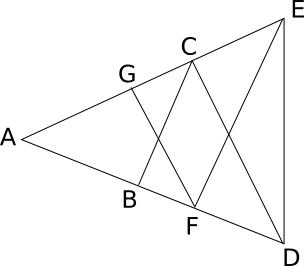Question 152

# In the figure AB=BC=CD=DE=EF=FG=GA, then $$\angle{DAE}$$ is approximatelySolution

So according to given conditions we get , ACB = x.

As an external angle CBD = 2x.

Also we know that total angle on an line is 180 we get EFD = 3x because of which EDF = 3x.

Similarly on opposite side we get AED = 3x.

So in total x+3x+3x = 180.

We get x = 25 (approx).